# How to Crack a Cheating Scandal with 1 Line of Code

## Explaining and Simulating Binomial Distributions in R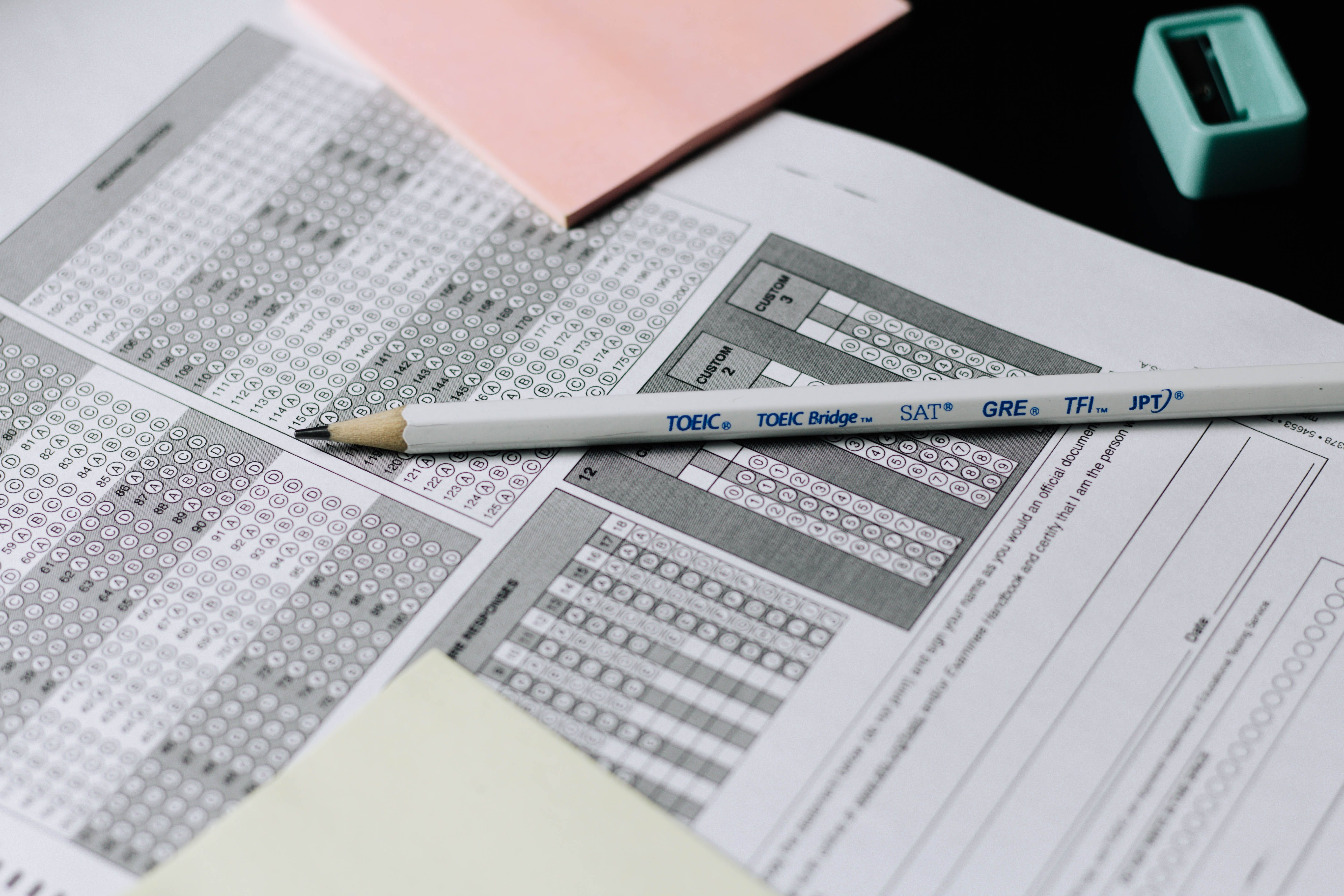Photo by Nguyen Dang Hoang Nhu on Unsplash
1. How cheating scandals and binomial distributions are related
2. How to simulate a binomial distribution (and something called the CDF) in R

## What’s a Binomial Distribution?

Okay, we’ll come back to your coin-flip fiasco in a moment, but first we need to talk about statistics.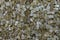Photo by Pawel Czerwinski on Unsplash
• Each trial has to have the same success rate. A coin does not have a 50% success rate on one flip, then suddenly switches to an 80% success rate on the next flip. The coin is consistent from trial to trial.
• Each trial has to be independent. This means that the result of one trial does not affect the outcome of another trial. If a coin lands on heads 5 times in a row, that does not change the probability of the coin landing on heads or tails on the 6th flip. A coin has no memory.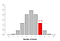Image by Author

## Cheating Scandals and Binomial Distributions

So that’s the binomial distribution, but are they useful in the real world?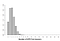Image by Author

## PMFs and CDFs

Okay, so we know what a binomial distribution is, and we know that binomial distributions can help unravel real cheating scandals, but how are we supposed to get your money back from Dave, the coin-flipping fiend? How do we actually show that he is suspiciously likely?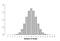Image by Author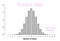Image by Author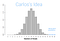Image by Author
• CDF (cumulative density function): gives the probability of getting an exact outcome or better/worse (18 or more heads out of 20)
• `pbinom(q,n,p)` — Calculates the CDF of a binomial distribution. Specifically, pbinom calculates the probability that the number of successes is less than or equal to q.
• `n `— trials in the binomial distribution
• `p` — probability of success in the binomial distribution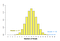Image by Author

## Conclusion

If you run the line of code for Dave’s coin-flipping:

A human, writing (mostly) about math | California | If you want to reach out beneschan@gmail.com